PHY294H - Lecture 25

Recommended problems: 31.45,31.46

Rotating loops - Electric Generators and motors

We will now show that if we rotate a loop at constant angular speed in a uniform and constant magnetic field, then we generate an oscillatory emf. In an electric generator, we rotate a loop through a magnetic field using a chemical or nuclear energy source. This produces the oscillatory emf we use in our society. An electric motor works in reverse. If we apply an oscillatory emf to a current loop in a magnetic field, it rotates at constant angular speed. Recall that in the case of a DC current source, we had to switch the current direction every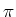rotation in order to keep the loop moving in the same direction. However in the case of an AC motor, the AC source switches polatity automatically everyrotation, so the direction of rotation of the loop is maintained.

Let us consider the situation in which a coil with N turns and area Ais rotated about its central axis at constant angular speed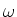. The angle between the magnetic moment of the loop and the applied magnetic field is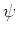. This angle increases as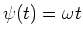due to the constant angular speed of the coil. The rate of change of the magnetic flux is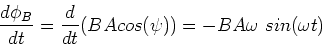(1)

The induced emf is given by Faraday's which gives,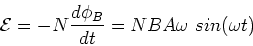(2)

The potential energy is given by,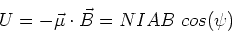(3)

The torque is given by,(4)

The mechanical power that must be supplied to rotate the coil is,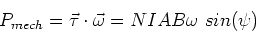(5)

This must be equal to the electrical power delivered to the load, this is given by,(6)

The average power dissipated is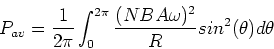(7)

Using the result that,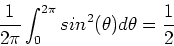(8)

we have,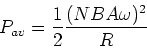(9)

which is the average power delivered by our generator. This is the number you see quoted on your lightbulb.